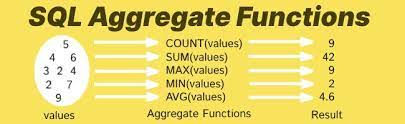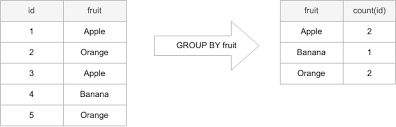top of pageSearch

# Aggregate Functions in SQL

SQL is used to communicate with a database. According to ANSI (American National Standards Institute), it is the standard language for relational database management systems.Structured query language (SQL) is a standard language for database creation and manipulation.

Functions in SQL

Functions in SQL Server are the database objects that contains a set of SQL statements to perform a specific task. A function accepts input parameters, perform actions, and then return the result. We should note that functions always return either a single value or a table.

Aggregate Functions

An aggregate function in SQL performs a calculation on multiple values and returns a single value. SQL provides many aggregate functions that include avg, count, sum, min, max, etc.

The following are the commonly used SQL aggregate functions:

• AVG() – returns the average of a set.

• COUNT() – returns the number of items in a set.

• MAX() – returns the maximum value in a set.

• MIN() – returns the minimum value in a set

• SUM() – returns the sum of all or distinct values in a set

Except for the COUNT() function, SQL aggregate functions ignore null.## SUM

The SUM() function returns the sum of all values. The following illustrates the syntax of the SUM() function

The COUNT() function returns the number of rows that matches a specified criterion.

The SUM() function returns the total sum of a numeric column.

### SUM() Syntax

SELECT SUM(column_name) FROM table_name WHERE condition;

For example, the following statement returns the total salary of all employees in each department:

```EXAMPLE:
SELECT
department_id, SUM(salary)
FROM
employees```

## COUNT

The COUNT() function returns the number of items in a set. The following shows the syntax of the COUNT() function.The COUNT(*)returns the total number of rows in a table, including the NULLs.

### COUNT() Syntax

SELECT COUNT(column_name) FROM table_name WHERE condition;

For example, the following example uses the COUNT(*) function to return the headcount of each department:

```EXAMPLE:
SELECT
FROM
employees```

Note: A column name of the table can also be used instead of *. Unlike COUNT(*), this variation COUNT(column) will not count NULLvalues in that column. Example: SELECT COUNT(employee_id) FROM employees WHERE experience < 5;

MAX

The MAX() function returns the maximum value of a set. The MAX() function has the following syntax:

### MAX() Syntax

SELECT MAX(column_name) FROM table_name WHERE condition;

For example, the following statement returns the highest salary of employees in each department:

```EXAMPLE:
SELECT
department_name, MAX(salary) highest_salary
FROM
employees```

## MIN

The MIN() function returns the minimum value of a set. The following illustrates the syntax of the MIN() function:

### MIN() Syntax

SELECT MIN(column_name) FROM table_name WHERE condition;

For example, the following statement returns the minimum salary of the employees in each department:

```EXAMPLE:
SELECT
department_name, MIN(salary) min_salary
FROM
employees```

## AVG

The AVG() function returns the average values in a set. The following illustrates the syntax of the AVG() function

The AVG() function returns the average value of a numeric column.

### AVG() Syntax

SELECT AVG(column_name) FROM table_name WHERE condition;

The following example shows how to use the AVG() function to calculate the average salary of each department:

```EXAMPLE:
SELECT
department_name, ROUND(AVG(salary), 0) avg_salary
FROM
employees

```

GROUP BY

The GROUP BY statement groups rows that have the same values into summary rows, like "find the number of customers in each country".Aggregate functions are often used with the GROUP BY clause of the SELECT statement.​

The GROUP BY statement is often used with aggregate functions (COUNT(), MAX(), MIN(), SUM(), AVG()) to group the result-set by one or more columns.

### GROUP BY Syntax

SELECT column_name(s) FROM table_name WHERE condition GROUP BY column_name(s) ORDER BY column_name(s);

EXAMPLE

SELECT item_id,item_name

FROM Market

WHERE item_name="fruit"

GROUP BY item_nameHAVING Clause

The HAVING clause was added to SQL because the WHERE keyword cannot be used with aggregate functions.

### HAVING Syntax

SELECT column_name(s) FROM table_name WHERE condition GROUP BY column_name(s) HAVING condition

EXAMPLE SELECT COUNT(CustomerID), Country

FROM Customers

GROUP BY Country

HAVING COUNT(CustomerID) > 5;

COMPARISON OF HAVING WITH GROUP BY AND WHERE CLAUSE

• A HAVING clause is like a WHERE clause, but applies only to groups as a whole (that is, to the rows in the result set representing groups), whereas the WHERE clause applies to individual rows. A query can contain both a WHERE clause and a HAVING clause.

• The “Group By” clause is used to group data into summary rows based on common values, while the “Having” clause is used to filter those groups based on a condition that applies to the aggregated data.

• The HAVING clause can contain aggregate funtion.​The GROUP BYClause cannotcontain aggregate funtion.​

• The HAVINGclause can filters groups, based on condition with aggregate function. But it can slow down query preformance in complex calculation.​

• The GROUP BY Clause can reduce the number of rows by grouping the same values and summerize data on multiple columns. But it is time consuming to write and difficult to understand the output of complex query.​

• The HAVING clause is used as extra condition to the Query.​

• The GROUP BYClause groups rows that have the same values into summary rows.​

## The SQL ORDER BY Keyword

The ORDER BY keyword is used to sort the result-set in ascending or descending order.

The ORDER BY keyword sorts the records in ascending order by default. To sort the records in descending order, use the DESC keyword.

### ORDER BY Syntax

SELECT column1, column2, ... FROM table_name ORDER BY column1, column2, ... ASC | DESC;

EXAMPLE

SELECT COUNT(CustomerID), Country

FROM Customers

GROUP BY Country

HAVING COUNT(CustomerID) > 5

ORDER BY COUNT(CustomerID) DESC;

Use the ORDER BY clause to display the output table of a query in either ascending or descending alphabetical order. Whereas the GROUP BY clause gathers rows into groups and sorts the groups into alphabetical order, ORDER BY sorts individual rows. The ORDER BY clause must be the last clause that you specify in a query.